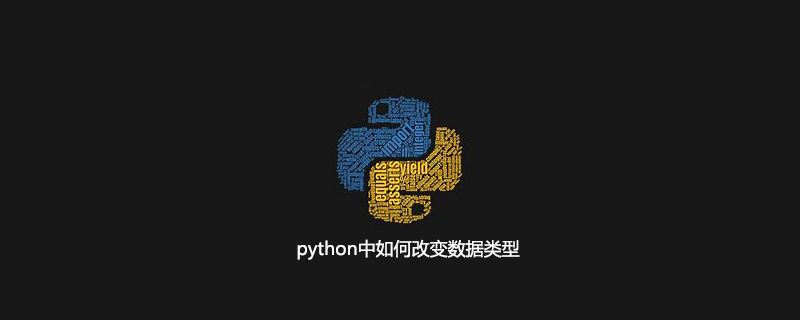Jquery中文网 www.jquerycn.cn
Jquery中文网 >  Python编程  >  Python入门  >  正文 python中如何改变数据类型

# python中如何改变数据类型

jquery中文网为您提供python中如何改变数据类型等资源，欢迎您收藏本站，我们将为您提供最新的python中如何改变数据类型资源python学习网，大量的免费python视频教程，欢迎在线学习！

1、虽然python中的变量不需要声明，但使用时必须赋值

（1）整形变量

（2）浮点型变量

（3）字符型

2、可以一个给多个变量赋值，也可以多个给多个变量赋值

3、python3中有6个标准数据类型

*Number(数字)

*True=1

*False=0

*数值的除法（/）总是返回一个浮点数，要获取整数使用//操作符

*在混合计算时，python会把整形转换为浮点数

*String(字符串)

*字符串用'或"括起来，同时使用\转义特殊字符串

*如果不想让反斜杠发生转义，可以在字符串前面加个r表示原始字符串

*索引值以0为开始，-1为末尾的开始位置

*加号 是字符串的连接符，星号*表示复制当前的字符串，紧跟的数字为复制的次数

*List(列表)

*list写在方括号之间，元素用逗号隔开

*和字符串一样，list可以被索引和切片

*list可以使用 操作符进行连接

*list中的元素可以改变的

*Tuple(元组)

*元组与列表类似，不同之处在于元组的元素不能修改，元组写在小括号里。元素之间用逗号隔开

*元组也可以被索引和切片，方法一样

*注意构造包含0或1个元素的元组的特殊语法规则

*元组也可以用 操作符进行拼接

*Sets(集合)

*set是一个无需不重复的序列，基本功能是进行成员关系的测试和删除重复元素

*Dictionary(字典)

*字典是一种映射类型，字典用{}标识，它是一个无序的建(key):值(value)对集合

*建(key)必须使用不可变类型。在同一个字典中建(key)必须是唯一的

*创建空字典使用{}

4、类型之间的转换

*int(x,base=10)x字符串或数字，base进制数，默认十进制，浮点转为整数

*float()整数转换为浮点型

*complex(1,2) 转换为复数

*str(10)将对象转换为字符串

*repe()将对象转换为表达式字符串

*repr(dict)将对象转换为表达式字符串

*eval(str)用来计算在字符串中有效的python表达式，返回一个对象

*tuple(listi)将列表转化为元组

*list()将元组转换为列表

*set转换集合

```print('------------------1----------------')
a=100#整形变量
b=100.0#浮点型变量
c='zifuxing'#字符串
print(a,b,c)
print('---------------------2------------------')
a=b=c=1
print(a,b,c)
a,b,c=1,2,3
print(a,b,c)
print('--------------------3-------------------')
print('Number 数字')
a,b,c=20,5.5,True
#type可以查询变量所指的数据类型
print(type(a),type(b),type(c))
#也可以用isinstance来判断
# type()不会认为子类是一种父类类型
#isinstance()会认为子类是一种父类类型
print('String 字符串')
str1='zifuchuan'
print(str1[0:-1])#输出第一个到倒数第二个
print(str1)#输出第一个字符串
print(str1[2:5])#输出第三个到第五个字符串
print(str1[2:])#输出第三个后面所有的字符串
print(str1*2)#输出字符串2次
print(str1 'Test')#链接字符串
print('列表')
listp=['abc',768,2.33,'liebiao',70.2]
tinylist=[123,'liebiao']
print(listp)#输出完整列表
print(listp)#输出列表的第一个元素
print(listp[1:3])#输出第二个元素到第三个元素
print(listp[2:])#输出第三个元素开始的所有元素
print(tinylist*2)#输出两次列表
print(listp tinylist)#链接两个列表
#该变列表中的元素
a=[1,2,3,4,5,6]
a=9
a[2:5]=[13,14,5]
a[2:5]=[]#可以删除所指定的元素
print('Tuple 元组,用法跟上面的一样但修改不了元素')
print('set 集合')
student={'Tom','Jim','Mary','Tom','Jack','Rose'}
print(student)#输出集合，重复的元素被自动去掉
if 'Rose' in student:
print('Rose 在集合中')
else:
print('Rose不在集合中')
#set可以进行集合运算
a=set('abra')
b=set('alac')
print(a)#set可以去重复所以输出啊a,b,r
print(a-b)#a和b的差
print(a|b)#a和b,的并集
print(a&b)#a和b的交集
print(a^b)#a和b不同时存在的元素
print('Dictionary 字典')
tinydict={'name':'runoob','code':'1','site':'www.runoob.com'}
print(tinydict)#输出完整的字典
print(tinydict.keys())#输出所有的建
print(tinydict.values())#输出所有的值
print('----数据类型转换--------')
print(int(3.6))#浮点数转换为整数
print(float(1))#整数转换为浮点数
print(float('123'))#字符串转为浮点数
print(complex(1,2))#整数为复数
print(complex('1'))#字符串为负数
print(str(dict))
i=int(10)
print(str(i))
print(repr(dict))
x=7
print(eval('3*x'))#可以操作字符串